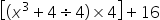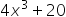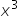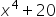Mathematics
Easy

Question

# What is the value of##20Hint:

## The correct answer is:### The given expression is [(x3 + 4 ÷ 4) × 4 ] + 16There is one variable expression. The variable is: ‘x’. We have to perform the operations between them and find the right answer.Let’s consider the inner bracket. In inner bracket there are two terms. x^3 and (4 ÷4)They are unlike terms as one is variable and second is constant. Unlike terms means they have different variables.The whole bracket is multiplied by 4 and then to the final value 16 is added.Let’s solve the expression step by step[(x3 + 4 ÷ 4) × 4 ] + 16We will have to follow the BODMAS rule. We have to follow the this order.B stands for bracket, O stands for order, D stands for division, M stands for multiplication, A stands for addition. And S stands for subtraction.So we will solve (4 ÷ 4) first[(x3 + 4 ÷ 4) × 4 ] + 16 = [( x3 + 1) × 4 ] + 16Now we will use distribute law here to distribute 4 for both the terms inside the bracket.Distributive property - The quantity outside the bracket, or in other words, quantity in multiplication with bracket is equally distributed to the terms inside the bracket. This property is used in the above equation. And this property is distributive property[(x2+ 4 ÷ 4) × 4 ] + 16 = ( x3 × 4 + 1 × 4)+ 16[(x3 + 4 ÷ 4) × 4 ] + 16 = ( 4x3 + 4)+ 16No further operation is possible for the bracket term as they are unlike terms.So we can write[(x3+ 4 ÷ 4) × 4 ] + 16 = 4x3+ 4 + 16[(x3+ 4 ÷ 4) × 4 ] + 16 = 4x3 + 20No further operation is possible as both are unlike terms.Therefore, the value of expression is 4x3 + 20So the option which is'4x3 + 20' is the right option.

We have to follow the proper order of operations, or we will get errors. We have to be careful about different types of variables.

### Related Questions to study#### With Turito Foundation.#### Get an Expert Advice From Turito.